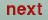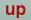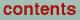Next: Spin polarized calculations Up: The STUTTGART TB-LMTO-ASA program. Previous: Accurate band structure away

# Frozen potential calculations

In the ASA it is easy to calculate for instance structural energy differences. This is done by the so-called frozen potential technique. Assume that we want to calculate the structural energy difference between fcc and bcc copper. First a self-consistent calculation for fcc copper is performed in the usual way and the total energy and a potential is obtained. The potential is inserted in the bcc structure with the same atomic volume and the total energy is calculated without iterating to self-consistency i.e. keeping the potential frozen. The total energy difference in the two calculations is the structural energy difference.

The second step is performed in the following way: The atomic file containing the frozen potential and potential parameters is not changed, but the CTRL file is.

In category START: ITER=1, so that only one iteration is performed and BEGMOM=F, so that the program will start by making the hamiltonian and overlap matrices. Eigenvalues and eigenvectors will be calculated and from the latter the moments are obtained.

In category START: NMIX=0 so that linear mixing is effective and BETA=1 to ensure that the moments are not mixed with the previous ones.

In category CLASS: IDMOD=2 for allchannels so that the E's are not shifted to the new center of gravity.

In category OPTIONS: NITATOM=0 so that only one incomplete loop in the atomic program is performed. This produces the contribution to the total energy from the sphere.

O. Jepsen
Thu Oct 12 14:48:45 MESZ 2000